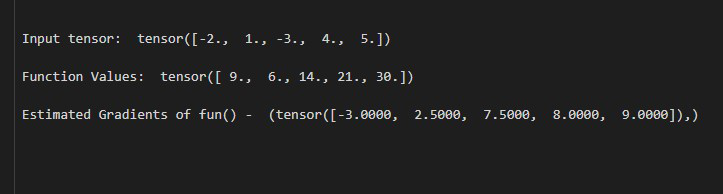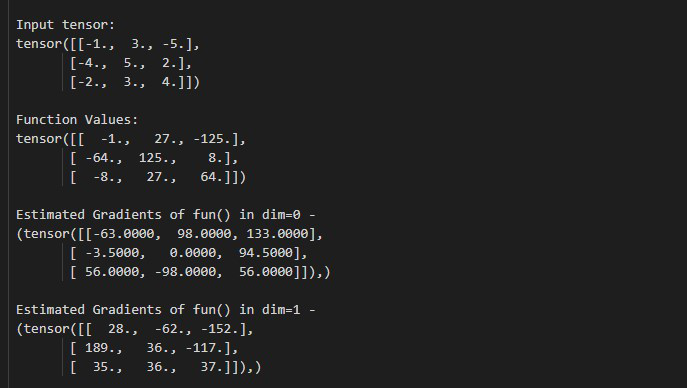Open In App

# How to Estimate the Gradient of a Function in One or More Dimensions in PyTorch?

In this article, we are going to see how to estimate the gradient of a function in one or more dimensions in PyTorch.

torch.gradient() method estimates the gradient of a function in one or more dimensions using the second-order accurate central differences method, and the function can be defined on a real or complex domain. For controllers and optimizers, gradient estimations are quite valuable. Gradient descent is a prominent optimization method that requires an estimate of the output derivatives with respect to each input at a given location. Let’s have a look at the syntax of the given method first:

Parameters:

• values(Tensor): this parameter is represents the values of the function.

### Example 1

In this example, we estimate the gradient of a function for a 1D tensor.

## Python3

 `# Import required library``import` `torch`` ` `# define the tensor``tens ``=` `torch.tensor([``-``2.``, ``1.``, ``-``3.``, ``4.``, ``5.``])``print``(``" Input tensor: "``, tens)`` ` `# define a function``def` `fun(tens):``    ``return` `tens``*``*``2``+``5`` ` `# values of function``values ``=` `fun(tens)`` ` `# display values``print``(``" Function Values: "``, values)`` ` `# estimate the gradients of fun``grad ``=` `torch.gradient(values)`` ` `# Display result``print``(``" Estimated Gradients of fun() - "``, grad)`

Output:### Example 2

In this example, we estimate the gradient of a function for a 2D tensor.

## Python3

 `# Import required library``import` `torch`` ` `# define the tensor``tens ``=` `torch.tensor([[``-``1.``, ``3.``, ``-``5.``],``                     ``[``-``4.``, ``5.``,  ``2.``],``                     ``[``-``2.``, ``3.``,  ``4.``], ])`` ` `print``(``"\n Input tensor: \n"``, tens)`` ` `# define a function``def` `fun(tens):``    ``return` `tens``*``*``3`` ` `# values of function``values ``=` `fun(tens)`` ` `# display values``print``(``"\n Function Values: \n"``, values)`` ` `# estimate the gradients of fun in dim=0``grad_dim_0 ``=` `torch.gradient(values, dim``=``0``)``print``(``"\n Estimated Gradients of fun() in dim=0 - \n"``, grad_dim_0)`` ` `# estimate the gradients of fun in dim=1``grad_dim_1 ``=` `torch.gradient(values, dim``=``1``)``print``(``"\n Estimated Gradients of fun() in dim=1 - \n"``, grad_dim_1)`

Output: